An organic compound C4H6 on ozonolysis give HCHO,CO2,CH3CHO.Compound will be:-
(a) H2C=CH-CH=CH2
(b) CH3-CH=C=CH2
(c) CH3-C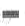C-CH3
(d)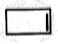Difficulty Level: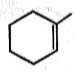$\underset{{\mathrm{H}}_{2}\mathrm{O}}{\overset{\mathrm{HCHO},{\mathrm{H}}^{+}}{\to }}$major product of this reaction is:

(a)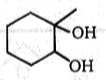(b)

(c)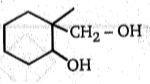(d)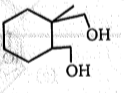High Yielding Test Series + Question Bank - NEET 2020

Difficulty Level: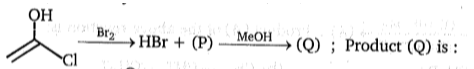(a)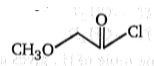(b)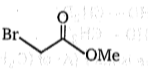(c)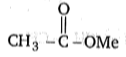(d)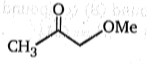High Yielding Test Series + Question Bank - NEET 2020

Difficulty Level:Product (C) of the reaction is:-

(a)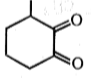(b)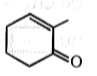(c)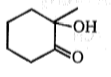(d)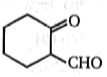High Yielding Test Series + Question Bank - NEET 2020

Difficulty Level:

What is the major product expected from the following reaction?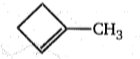$\stackrel{\mathrm{D}-\mathrm{Cl}}{\to }$ Product

1.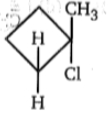2.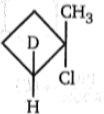3.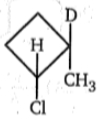4.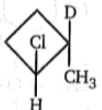High Yielding Test Series + Question Bank - NEET 2020

Difficulty Level: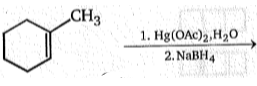Product; Product is:-

(a)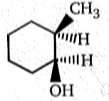(b)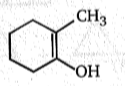(c)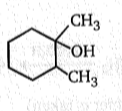(d)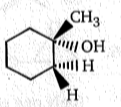High Yielding Test Series + Question Bank - NEET 2020

Difficulty Level:

How many stereoisomeric tetrabromides will be formed in the following reaction  ?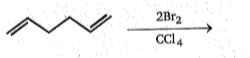(a)2 (b)3 (c) 4 (d) 6

Difficulty Level:

${\mathrm{CH}}_{3}-\underset{|}{\mathrm{C}}\mathrm{H}-{\mathrm{CO}}_{2}\mathrm{K}\phantom{\rule{0ex}{0ex}}{\mathrm{CH}}_{3}-\mathrm{CH}-{\mathrm{CO}}_{2}\mathrm{K}$ $\stackrel{\mathrm{electrolysis}}{\to }$ (A) (Major)

Major product (A) of the above reaction: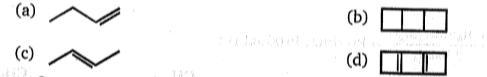High Yielding Test Series + Question Bank - NEET 2020

Difficulty Level: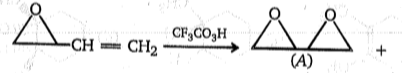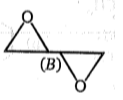(Only one enantiomer is taken)
Which of the following statement is correct about A and B ?
(a) A  and B are mixture of diastereomers
(b) A and B are mixture of enantiomers
(c) A and B are optically active
(d) B is racemic mixture

High Yielding Test Series + Question Bank - NEET 2020

Difficulty Level: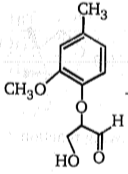$\stackrel{{\mathrm{NaBH}}_{4}}{\to }$A$\stackrel{{\mathrm{O}}_{3}}{\to }$B$\stackrel{{\mathrm{H}}_{2}\mathrm{O}}{\to }$(c) (one of the product)

Identify the product (C):

(a) $\begin{array}{ccccccc}{\mathrm{CH}}_{3}& -& \stackrel{\mathrm{O}}{\stackrel{\parallel }{\mathrm{C}}}& -& \stackrel{\mathrm{O}}{\stackrel{\parallel }{\mathrm{C}}}& -& \mathrm{O}\begin{array}{cc}-& {\mathrm{CH}}_{3}\end{array}\end{array}$

(b) $\begin{array}{ccc}\underset{|}{{\mathrm{CH}}_{2}}& -& \mathrm{OH}\\ \underset{|}{\mathrm{CH}}& -& \mathrm{OH}\\ {\mathrm{CH}}_{2}& -& \mathrm{OH}\end{array}$

(c) $\begin{array}{ccc}\stackrel{\mathrm{CHO}}{\stackrel{|}{\underset{|}{\mathrm{CH}}}}& -& \mathrm{OH}\\ {\mathrm{CH}}_{2}& -& \mathrm{OH}\end{array}$

(d) $\begin{array}{c}\underset{|}{\mathrm{CHO}}\\ \underset{|}{\mathrm{CHOH}}\\ \mathrm{CHO}\end{array}$